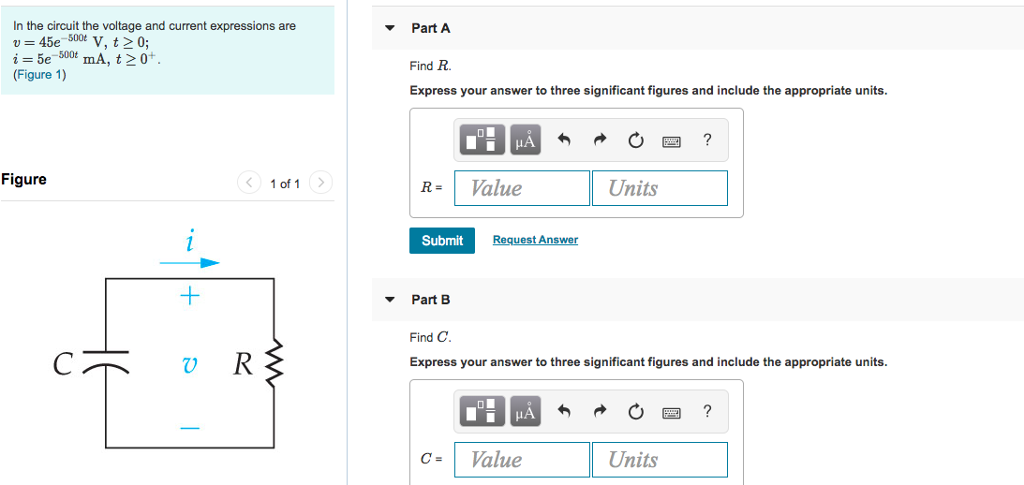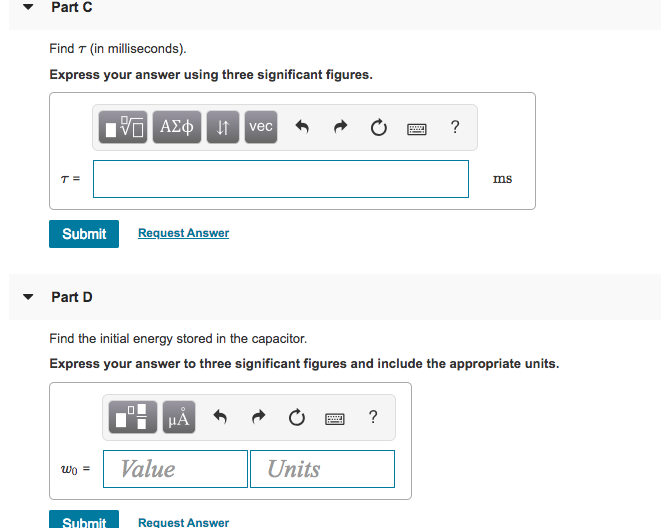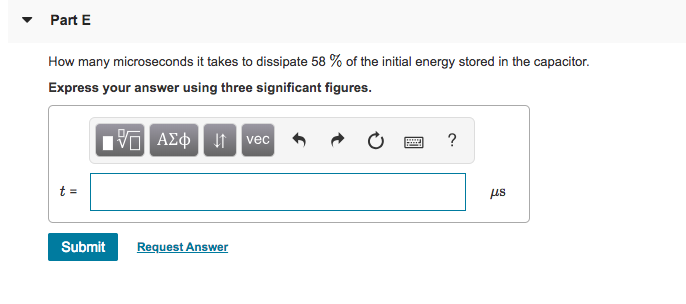# In the circuit the voltage and current expressions are ▼ Part A Find R Express your...

###### Question:In the circuit the voltage and current expressions are ▼ Part A Find R Express your answer to three significant figures and include the appropriate units. Figure 1) Figure < 1of1 > RValue Units Submit Part B Find C Express your answer to three significant figures and include the appropriate units. c-Value Units